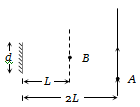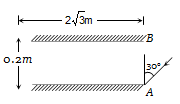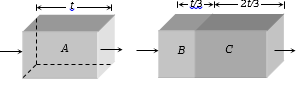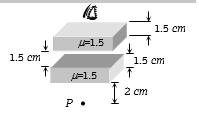A point source of light B is placed at a distance L in front of the centre of a mirror of width d hung vertically on a wall. A man walks in front of the mirror along a line parallel to the mirror at a distance 2L from it as shown. The greatest distance over which he can see the image of the light source in the mirror is(1) d/2

(2) d

(3) 2d

(4) 3d

Subtopic:  Reflection at Plane Surface |
To view explanation, please take trial in the course below.
NEET 2022 - Target Batch - Aryan Raj Singh

Difficulty Level:

Two plane mirrors, A and B are aligned parallel to each other, as shown in the figure. A light ray is incident at an angle of $30°$ at a point just inside one end of A. The plane of incidence coincides with the plane of the figure. The maximum number of times the ray undergoes reflections (excluding the first one) before it emerges out is :1.  28

2.  30

3.  32

4.  34

Subtopic:  Reflection at Plane Surface |
To view explanation, please take trial in the course below.
NEET 2022 - Target Batch - Aryan Raj Singh

Difficulty Level:

A concave mirror of focal length 100 cm is used to obtain the image of the sun which subtends an angle of $30°$. The diameter of the image of the sun will be

(1)

(2) $0.87cm$

(3)

(4) 100 cm

Subtopic:  Reflection at Spherical Surface |
To view explanation, please take trial in the course below.
NEET 2022 - Target Batch - Aryan Raj Singh

Difficulty Level:

A square of side 3 cm  is placed at a distance of 25 cm from a concave mirror of focal length 10 cm. The centre of the square is at the axis of the mirror and the plane is normal to the axis. The area enclosed by the image of the square is

(1)

(2)

(3)

(4)

Subtopic:  Reflection at Spherical Surface |
To view explanation, please take trial in the course below.
NEET 2022 - Target Batch - Aryan Raj Singh

Difficulty Level:

A ray of light falls on the surface of a spherical glass paperweight making an angle $\alpha$ with the normal and is refracted in the medium at an angle $\beta$ . The angle of deviation of the emergent ray from the direction of the incident ray

(a) $\left(\alpha -\beta \right)$                     (b) $2\left(\alpha -\beta \right)$

(c) $\left(\alpha -\beta \right)/2$                 (d) $\left(\beta -\alpha \right)$

Subtopic:  Refraction at Curved Surface |
To view explanation, please take trial in the course below.
NEET 2022 - Target Batch - Aryan Raj Singh

Difficulty Level:

Light enters at an angle of incidence in a transparent rod of refractive index n. For what value of the refractive index of the material of the rod the light once entered into it will not leave it through its lateral face what so ever be the value of angle of incidence

(1) $n>\sqrt{2}$

(2) $n=1$

(3) $n=1.1$

(4) $n=1.3$

Subtopic:  Total Internal Reflection |
To view explanation, please take trial in the course below.
NEET 2022 - Target Batch - Aryan Raj Singh

Difficulty Level:

One face of a rectangular glass plate 6 cm thick is silvered. An object held 8 cm in front of the first face, forms an image 10 cm behind the silvered face. The refractive index of the glass is [Consider that light ray returns back in the first medium]

(1) 0.4

(2) 0.8

(3) 1.5

(4) 1.6

Subtopic:  Refraction at Plane Surface |
To view explanation, please take trial in the course below.
NEET 2022 - Target Batch - Aryan Raj Singh

Difficulty Level:

Two transparent slabs have the same thickness as shown. One is made of material A of refractive index 1.5. The other is made of two materials B and C with thickness in the ratio 1:2. The refractive index of C is 1.6. If a monochromatic parallel beam passing through the slabs has the same number of waves inside both, the refractive index of B is1.  1.1

2.  1.2

3.  1.3

4.  1.4

Subtopic:  Refraction at Plane Surface |
To view explanation, please take trial in the course below.
NEET 2022 - Target Batch - Aryan Raj Singh

Difficulty Level:

The image of point P when viewed from the top of the slabs will be(1)   2.0 cm above P

(2)   1.5 cm above P

(3)   2.0 cm below P

(4)   1 cm above P

Subtopic:  Refraction at Plane Surface |
To view explanation, please take trial in the course below.
NEET 2022 - Target Batch - Aryan Raj Singh

Difficulty Level:

Two point light sources are 24 cm apart. Where should a convex lens of focal length 9 cm be put in between them from one source so that the images of both the sources are formed at the same place?

(1)   6 cm

(2)   9 cm

(3)   12 cm

(4)   15 cm

Subtopic:  Lenses |
To view explanation, please take trial in the course below.
NEET 2022 - Target Batch - Aryan Raj Singh

Difficulty Level: